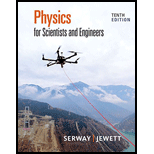Chapter 16, Problem 42PPhysics for Scientists and Enginee...

10th Edition
Raymond A. Serway + 1 other
ISBN: 9781337553278

Solutions

Chapter
SectionPhysics for Scientists and Enginee...

10th Edition
Raymond A. Serway + 1 other
ISBN: 9781337553278
Textbook Problem

Review. A block with a speaker bolted to it is connected to a spring having spring constant k and oscillates as shown in Figure P16.41. The total mass of the block and speaker is m, and the amplitude of this unit’s motion is A. The speaker emits sound waves of frequency f. Determine (a) the highest and (b) the lowest frequencies heard by the person to the right of the speaker. (c) If the maximum sound level heard by the person is β when the speaker is at its closest distance d from him, what is the minimum sound level heard by the observer?

(a)

To determine

The highest frequency heard by person to the right of the speaker.

Explanation

The spring constant is k, the total mass of the block and the speaker is m, the amplitude of the unit’s motion is d and the frequency of the sound wave is f.

Formula to calculate the highest frequency when speaker is moving towards person is,

fmax=vsv0vsvmaxf        (1)

Here, vs is the speed of sound, vmax is the maximum speed, f is the frequency of the sound wave and fmax is the highest frequency when speaker is moving towards person.

Since, person is at rest but the speaker is moving. Hence, the speed of the observer is zero.

Substitute 0 for v0 in equation (II) to find the fmax

(b)

To determine

The lowest frequency heard by person to the right of the speaker.

(c)

To determine

The minimum sound level heard by the observer.

Still sussing out bartleby?

Check out a sample textbook solution.

See a sample solution

The Solution to Your Study Problems

Bartleby provides explanations to thousands of textbook problems written by our experts, many with advanced degrees!

Get Started

Complete each of the following reactions: a. b.

Chemistry for Today: General, Organic, and Biochemistry

Describe the three ways thermal energy can be transported.

Foundations of Astronomy (MindTap Course List)

What is meant by residence time? Does seawater itself have a residence time?

Oceanography: An Invitation To Marine Science, Loose-leaf Versin